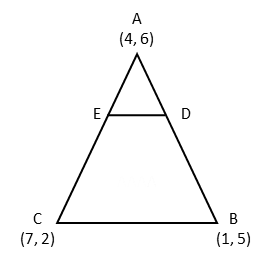# CBSE 2016 Maths Paper

10th board paper Q28. Coordinate Geometry

#### Question: In the figure below, the vertices of ∆ ABC are A (4, 6), B(1, 5) and C (7, 2). A line -segment DE is drawn to intersect the sides AB and AC at D and E respectively such that $$frac{AD}{AB}$ = $\frac{AE}{AC}$ = $\frac{1}{3}$ calculate the area of ∆ ADE and compare it with area of ∆ABC.#### Video Explanation #### Explanatory AnswerLet us find out coordinate of points D and E. AD : DB = 1 : 2 and AE : EC = 1 : 2 Coordinates of D = $$ $frac {2×4+1×1} {2+1}$,$\frac {2×6+1×5} {2+1}$ ) = $$$frac{9}{3}$, $\frac{17}{3}$) =$3, $$frac{17}{3}$) Coordinates of E = $$$frac{2 × 4 + 1 ×7}{3}$, $\frac{2 × 6 + 1 ×2}{3}$) =$5, $$frac{14}{3}$) Area of a triangle whose coordinates are$x1, y1), (x2, y2), and (x3, y3)
= $$frac{1}{2}$ {x1$y2 - y3 ) - x2 (y1 - y3) + x3 (y1 - y2)}

Area of ADE whose coordinates are (4, 6) , (3, $$frac{17}{3}$) and$5, $$frac{14}{3}$) = $\frac{1}{2}$ {4 $$$frac{17}{3}$ - $\frac{14}{3}$) - 3$6 - $$frac{14}{3}$) + 5$6 - $$frac{17}{3}$)} = $\frac{1}{2}$ {4 × 1 - 3 × $\frac{4}{3}$ + 5 × $\frac{1}{3}$} = $\frac{5}{6}$ square units Area of triangle ABC whose coordinates are$4, 6), (1, 5), and (7, 2)
= $$frac{1}{2}$ {4$5 - 2) - 1 (6 - 2 ) + 7 (6 - 5)}
= $\frac{1}{2}$ {4 × 3 - 4 + 7} = $\frac{15}{2}$

$\frac{Area of ∆ ABC}{Area of ∆ ABE}$ = $\frac{15/2}{5/6}$
= $\frac{15}{2}$ × $\frac{6}{5}$ = 9.
Sides of ADE = $\frac{1}{3}$ Sides of ABC
Area of ADE = $\frac{1}{9}$ Area of ABC